Get instant live expert help with Excel or Google Sheets“My Excelchat expert helped me in less than 20 minutes, saving me what would have been 5 hours of work!”

#### Post your problem and you’ll get Expert help in seconds.

Your message must be at least 40 characters
Our professional Expert are available now. Your privacy is guaranteed.

# Extract date from a date and time

Dates are cleverly stored as serial numbers while times are stored as decimal values relative to 24 hours in one day.  While working with Excel, we can extract only the date portion by using the INT or TRUNC function. This step by step tutorial will assist all levels of Excel users in extracting date from a date and time using two methods.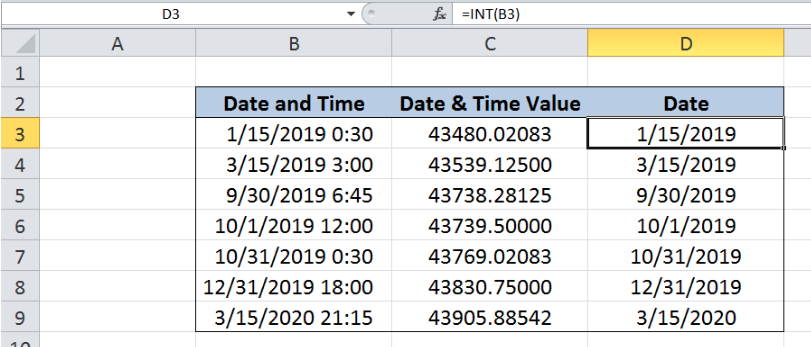Figure 1.  Final result: Extract date from date and time

Formula 1: `=INT(B3)`

Formula 2: `=TRUNC(B3)`

## Syntax of INT Function

INT rounds a number down to the nearest integer

`=INT(number)`

• number – the number we want to round down to the nearest integer

## Syntax of TRUNC Function

TRUNC returns an integer by removing the fractional part of the number

`=TRUNC(number, [num_digits])`

• number  – the number we want to truncate
• num_digits  – Optional;  a number that represents the digits after the decimal  point specifying the precision of the truncation; If omitted, the default value for num_digits is 0 (zero).

TRUNC and INT are similar such that both functions return integers.  TRUNC removes the fraction or decimal part of the number while INT rounds down the numbers to the nearest integer.

## Setting up our Data

Our table consists of three columns: Date and Time (column B), Date & Time Value (column C) and Date (column D).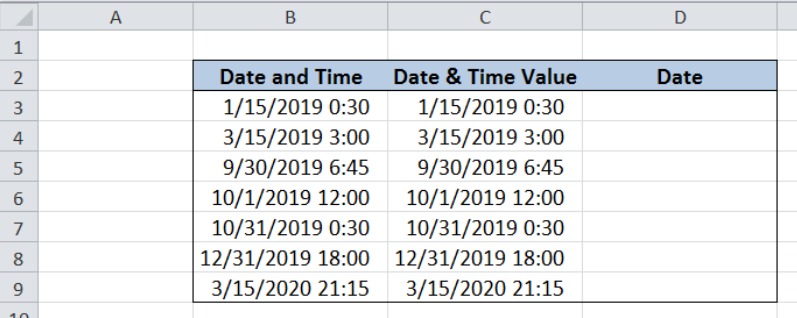Figure 2.  Sample date to extract date from date and time

The values in column B and C are the same, and column C is not needed in our formula.  However, we only want to show here how Excel stores date and time through numbers. Let us display the value of the date and time by following these steps:

Step 1.  Select cells C3:C9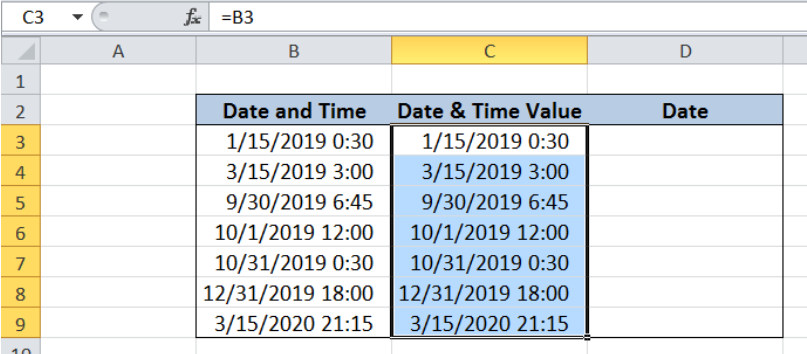Figure 3.  Selecting C3:C9 to change format to numbers

Step 2.  Press Ctrl + 1  to launch the Format Cells dialog box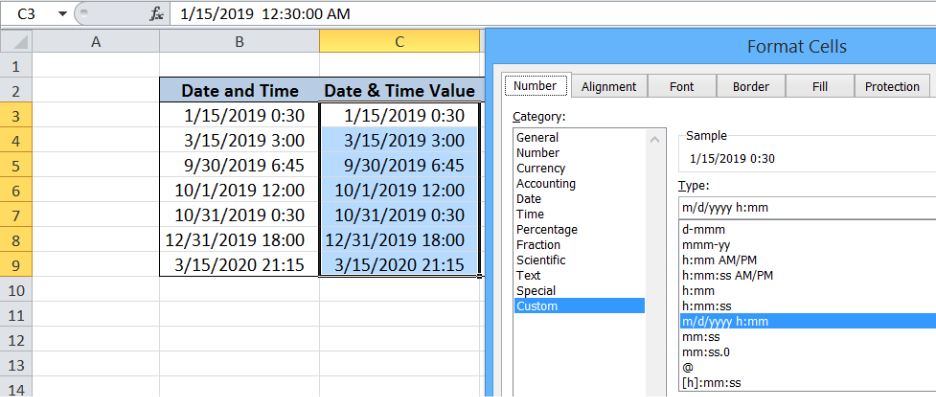Figure 4.  Format Cells preview

Step 3.  Change the format to Number, with 5 decimal places.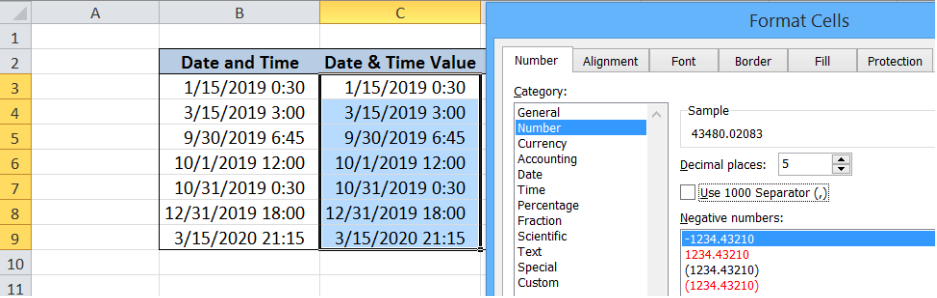Figure 5.  Changing the format to Number with 5 decimal places

Step 4.  Click OK.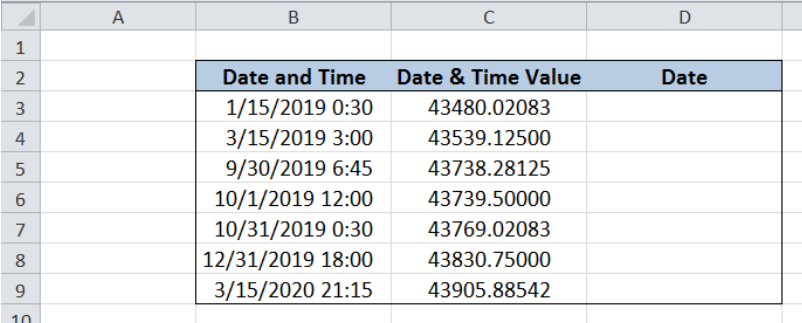Figure 6.  Column C showing values of date and time

We now have a table showing the values in column C.  Note that the whole numbers represent the serial number for the date, while the decimal values represent the time.

Dates have serial numbers where the value 1 corresponds to the starting date January 1, 1900, and each succeeding day is incremented by one.  One whole day has the value of 1 and one hour is equivalent to 1/24, since there are 24 hours in one day.

We want to extract the date from the date and time.  This can be done by extracting only the whole numbers or integers, through the INT or TRUNC function.

## Extract date from a date and time using INT

In order to extract the integers that correspond to the date, we will be using the INT function through these steps:

Step 1.  Select cell D3

Step 2.  Enter the formula: `=INT(B3)`

Step 3.  Press ENTER

The INT function rounds down any number into the nearest integers.  We have now extracted only the whole number or integers by using INT function.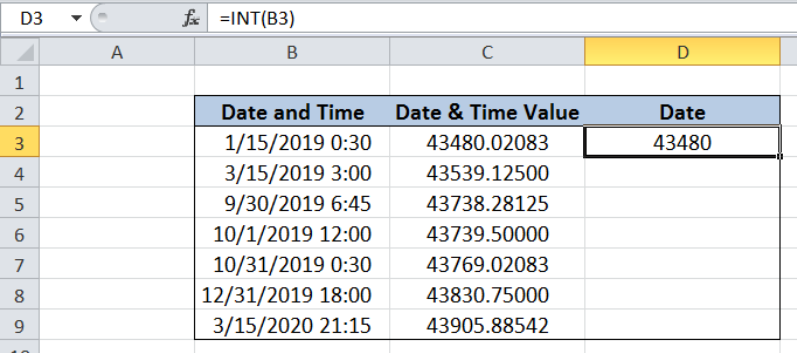Figure 7.  Extracting serial number only from date and time using INT

Step 4.  Format the cell to show the date by pressing Ctrl + 1

Step 5.  Select the category Date and the Type: d/mm/yyyy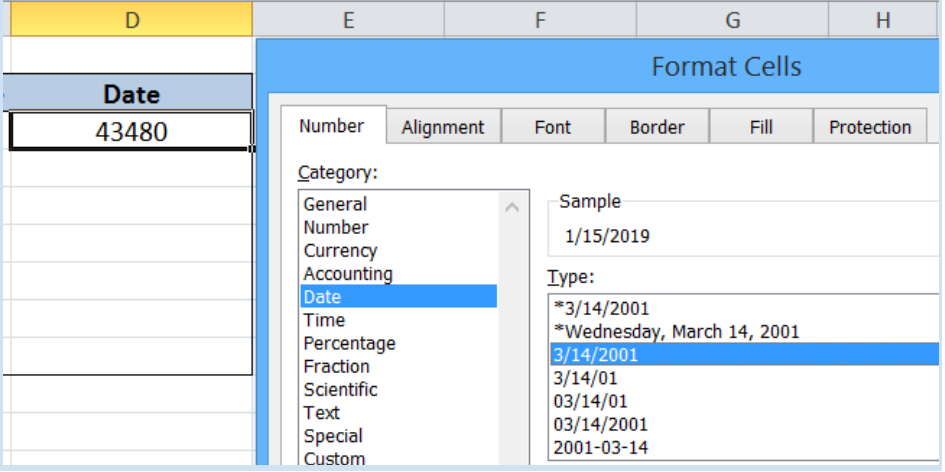Figure 8.  Changing format to show date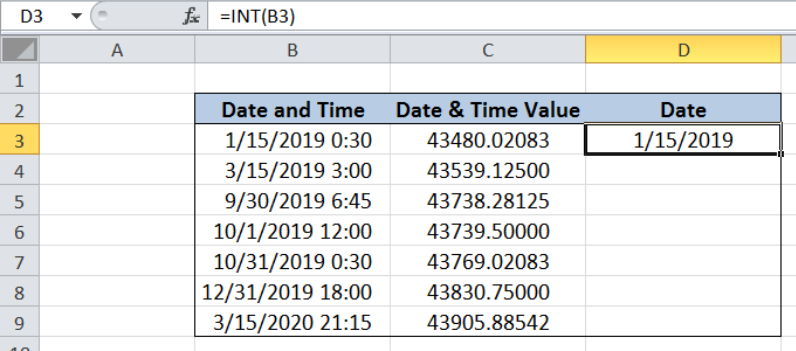Figure 9.  Display changed from serial number to date

Step 6.  Copy the formula in D3 to cells D4:D9 by clicking the “+” icon at the bottom-right corner of cell D3 and dragging it down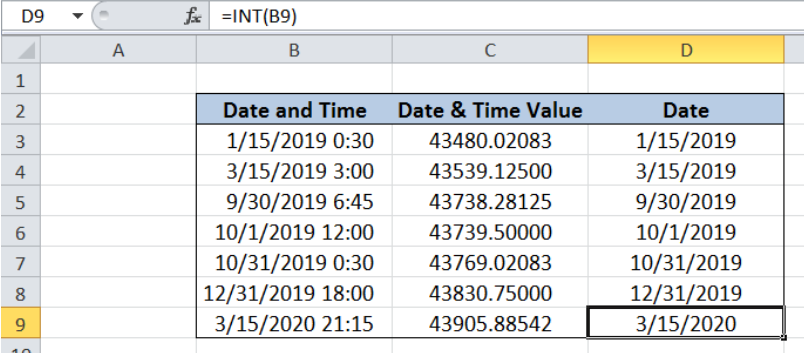Figure 10.  Output: Extract date from date and time using INT

## Extract date from a date and time using TRUNC

An alternative method to extract the date from a date and time is by using the TRUNC function.  The procedure is the same as in the previous method, but we only replace the function with TRUNC. Our working formula becomes:

`=TRUNC(B3)`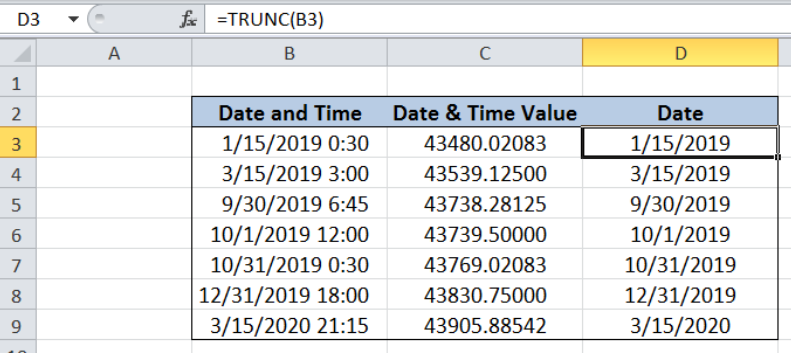Figure 11. Output: Extract date from date and time using TRUNC

Most of the time, the problem you will need to solve will be more complex than a simple application of a formula or function. If you want to save hours of research and frustration, try our live Excelchat service! Our Excel Experts are available 24/7 to answer any Excel question you may have. We guarantee a connection within 30 seconds and a customized solution within 20 minutes.

### Did this post not answer your question? Get a solution from connecting with the expert.Another blog reader asked this question today on Excelchat:
Solution examplesI have 2 cells containing a % value. The value for both is 9%. I want to conditionally format these cells as duplicate values but excel only highlights them as duplicate values if the decimal point is exactly the same. How can i correct this?
Solved by S. C. in 40 minsI need help with this formula. I need this to include months =INT((M4)/7)&" weeks"&IF(MOD(M4,7)=0,"",", "&INT(MOD(M4,7))&" days") I have the number of Days to Start with
Solved by F. A. in 29 minsI WANT TO WRITE A FORMULA THAT SAYS IF THE NUMBER IN COLUMN A MATCHES THE NUMBER IN COLUMN B THE INSERT THE NUMBER FROM COLUMN C INTO COLUMN D
Solved by A. W. in 20 minsExcel formula returns a lot of #N/A results but unsure how to incorporate the IFERROR function to remove them, Can anybody help? =IF(\$A3="","",IF(VLOOKUP(\$A3,'SHAREPOINT LINK]Jobs Log'!\$A:\$Y,17,FALSE)="","",VLOOKUP(\$A3,'SHAREPOINT LINK]Jobs Log'!\$A:\$Y,17,FALSE))) I have removed the link to the sharepoint doc as it gives away the company name so where it says 'SHAREPOINT LINK' just ignore, I don't think anyone needs to know that info. to get the formula to work. Thanks in advance to anyone able to help.
Solved by X. E. in 5 minsi need a formula to calculate previous day intraday high from google finance to google sheet. I AM USING THIS ONE =GOOGLEFINANCE(A2,"high",today()-1) BUT ITS NOT WORKING AND GIVING ME ERROR "When evaluating GOOGLEFINANCE, the query for the symbol: 'HDFCBANK' returned no data." HERE A2 IS "HDFCBANK" A TICKER.. AND I ALSO DONT WANT DATE COLUMN WITH THE PREVIOUS DAY INTRADAY HIGH PRICE.
Solved by G. E. in 40 mins## Subscribe to Excelchat.coAnother blog reader asked this question today on Excelchat: